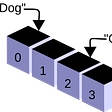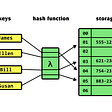# Recursion

`class Inception{  function KeepDreaming(){    //Do something        KeepDreaming();   }}`
`function fibonacciIterative(n){  let arr = [0, 1];  for (let i = 2; i < n + 1; i++){    arr.push(arr[i - 2] + arr[i -1]);  } return arr[n];}fibonacciIterative(3);function fibonacciRecursive(n) {  if (n < 2){    return n;  }  return fibonacciRecursive(n - 1) + fibonacciRecursive (n - 2)}fibonacciRecursive(6)`

# Bubble Sort

`const numbers = [99, 44, 6, 2, 1, 5, 63, 87, 283, 4, 0];function bubbleSort(array) {  const length = array.length;  for (let i = 0; i < length; i++) {    for (let j = 0; j < length; j++) {       if(array[j] > array[j+1]) {        //Swap the numbers        let temp = array[j]        array[j] = array[j+1];        array[j+1] = temp;      }    }          }}bubbleSort(numbers);console.log(numbers);`

# Selection Sort

`const numbers = [99, 44, 6, 2, 1, 5, 63, 87, 283, 4, 0];function selectionSort(array) {  const length = array.length;  for(let i = 0; i < length; i++){    // set current index as minimum    let min = i;    let temp = array[i];    for(let j = i+1; j < length; j++){      if (array[j] < array[min]){        //update minimum if current is lower that what we had previously        min = j;      }    }    array[i] = array[min];    array[min] = temp;  }  return array;}selectionSort(numbers);console.log(numbers);`

# Insertion Sort

`const numbers = [99, 44, 6, 2, 1, 5, 63, 87, 283, 4, 0];function insertionSort(array) {  const length = array.length;  for (let i = 0; i < length; i++) {    if (array[i] < array) {      //move number to the first position      array.unshift(array.splice(i,1));    } else {      // only sort number smaller than number on the left of it. This is the part of insertion sort that makes it fast if the array is almost sorted.      if (array[i] < array[i-1]) {        //find where number should go        for (var j = 1; j < i; j++) {          if (array[i] >= array[j-1] && array[i] < array[j]) {            //move number to the right spot            array.splice(j,0,array.splice(i,1));          }        }      }    }  }}insertionSort(numbers);console.log(numbers);`

# Merge Sort

`const numbers = [99, 44, 6, 2, 1, 5, 63, 87, 283, 4, 0];function mergeSort (array) {  if (array.length === 1) {    return array  }  // Split Array in into right and left  const length = array.length;  const middle = Math.floor(length / 2)  const left = array.slice(0, middle)   const right = array.slice(middle)    return merge(    mergeSort(left),    mergeSort(right)  )}function merge(left, right){  const result = [];  let leftIndex = 0;  let rightIndex = 0;  while(leftIndex < left.length &&         rightIndex < right.length){     if(left[leftIndex] < right[rightIndex]){       result.push(left[leftIndex]);       leftIndex++;     } else{       result.push(right[rightIndex]);       rightIndex++    }  }    return result.concat(left.slice(leftIndex)).concat(right.slice(rightIndex));}const answer = mergeSort(numbers);console.log(answer);`

# Quick sort

`const numbers = [99, 44, 6, 2, 1, 5, 63, 87, 283, 4, 0];function quickSort(array, left, right){  const len = array.length;   let pivot;  let partitionIndex;  if(left < right) {    pivot = right;    partitionIndex = partition(array, pivot, left, right);        //sort left and right    quickSort(array, left, partitionIndex - 1);    quickSort(array, partitionIndex + 1, right);  }  return array;}   function partition(array, pivot, left, right){  let pivotValue = array[pivot];  let partitionIndex = left;  for(let i = left; i < right; i++) {    if(array[i] < pivotValue){      swap(array, i, partitionIndex);      partitionIndex++;    }  }  swap(array, right, partitionIndex);  return partitionIndex;}function swap(array, firstIndex, secondIndex){    var temp = array[firstIndex];    array[firstIndex] = array[secondIndex];    array[secondIndex] = temp;}//Select first and last index as 2nd and 3rd parametersquickSort(numbers, 0, numbers.length - 1);console.log(numbers);`

# Traversel Techniques — BFS vs DFS

--

--

--

## More from M Adnan A

Love writing, learning, sharing and love being sarcastic :)

Love podcasts or audiobooks? Learn on the go with our new app.

## Embedding an AWS QuickSight dashboard in a Rails application## CI/CD on the Edge — Part1## 🔹Sunday tournament is over!🔹## test IO Shares Software Testing Insights in Latest White Paper## Running Product at Brex## How to merge different authors in GitLove writing, learning, sharing and love being sarcastic :)

## 4-in-1 Priority First Search in Python: BFS, DFS, Prim’s, and Dijkstra’s algorithms## Arrays Data Structures Explained## [Algorithm Interview] Hash Table